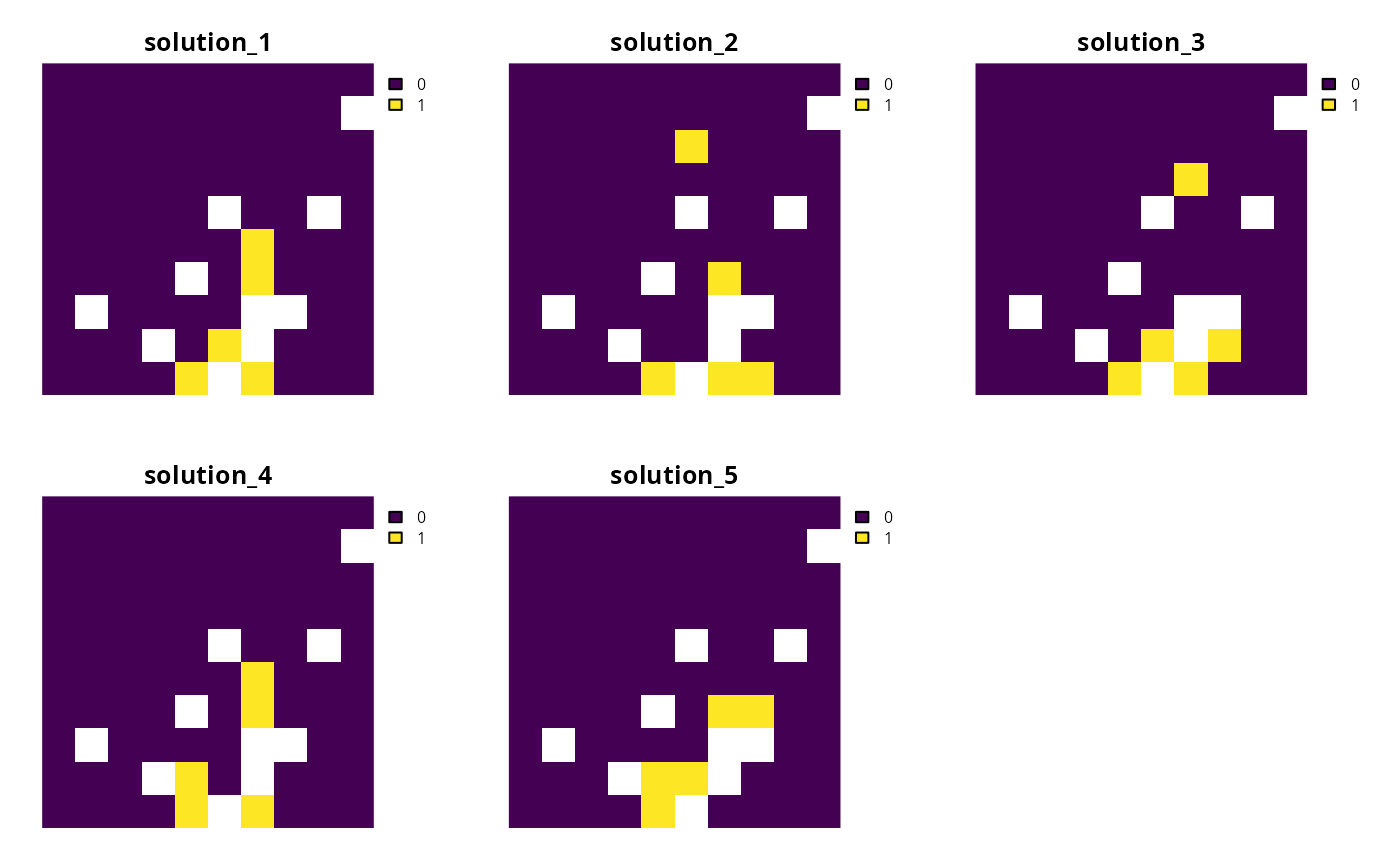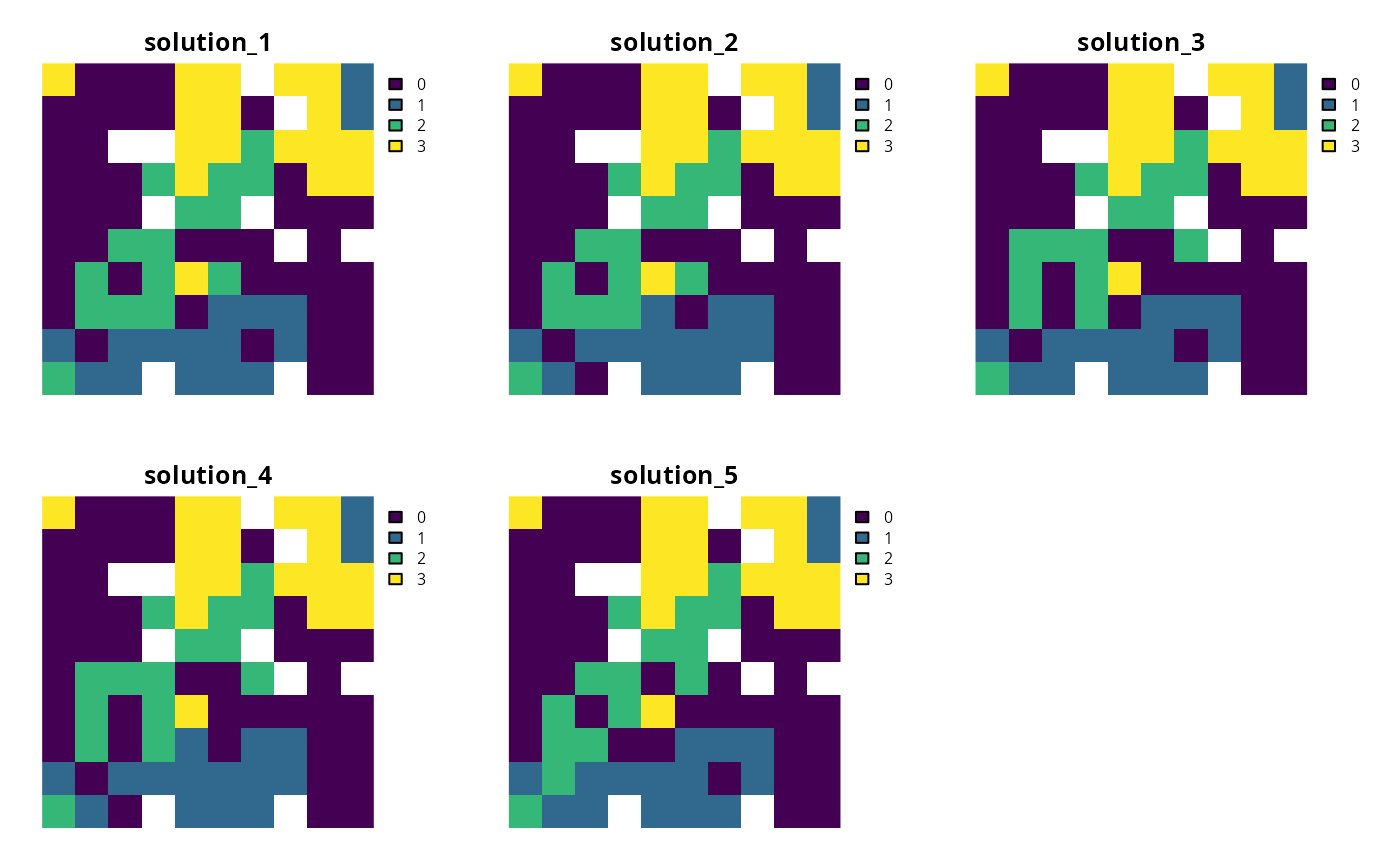Generate a portfolio of solutions for a conservation planning problem by finding a pre-specified number of solutions that are closest to optimality (i.e, the top solutions).

## Usage

add_top_portfolio(x, number_solutions = 10)

## Arguments

x

problem() object.

number_solutions

integer number of solutions required. Defaults to 10.

## Value

An updated problem() object with the portfolio added to it.

## Details

This strategy for generating a portfolio requires problems to be solved using the Gurobi software suite (i.e., using add_gurobi_solver(). Specifically, version 8.0.0 (or greater) of the gurobi package must be installed. Note that the number of solutions returned may be less than the argument to number_solutions, if the total number of feasible solutions is less than the number of solutions requested.

See portfolios for an overview of all functions for adding a portfolio.

Other portfolios: add_cuts_portfolio(), add_extra_portfolio(), add_gap_portfolio(), add_shuffle_portfolio()

## Examples

# \dontrun{
# set seed for reproducibility
set.seed(600)

sim_pu_raster <- get_sim_pu_raster()
sim_features <- get_sim_features()
sim_zones_pu_raster <- get_sim_zones_pu_raster()
sim_zones_features <- get_sim_zones_features()

# create minimal problem with a portfolio for the top 5 solutions
p1 <-
problem(sim_pu_raster, sim_features) %>%
add_default_solver(gap = 0, verbose = FALSE)

# solve problem and generate portfolio
s1 <- solve(p1)

# convert portfolio into a multi-layer raster
s1 <- terra::rast(s1)

# print number of solutions found
print(terra::nlyr(s1))
#>  5

# plot solutions
plot(s1, axes = FALSE)# create multi-zone problem with a portfolio for the top 5 solutions
p2 <-
problem(sim_zones_pu_raster, sim_zones_features) %>%
add_relative_targets(matrix(runif(15, 0.1, 0.2), nrow = 5, ncol = 3)) %>%
add_default_solver(gap = 0, verbose = FALSE)

# solve problem and generate portfolio
s2 <- solve(p2)

# convert each solution in the portfolio into a single category layer
s2 <- terra::rast(lapply(s2, category_layer))

# print number of solutions found
print(terra::nlyr(s2))
#>  5

# plot solutions in portfolio
plot(s2, axes = FALSE)# }